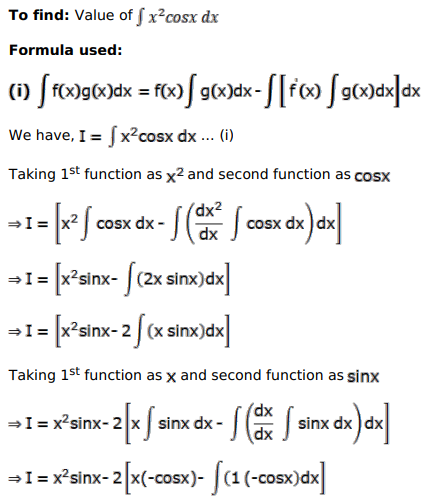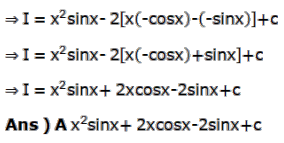# Mark against the correct answer in each of the following:

Question:

Mark $(\sqrt{)}$ against the correct answer in each of the following:

$\int x^{2} \cos x d x=?$

A. $x^{2} \sin x+2 x \cos x-2 \sin x+C$

B. $2 x \cos x-x \sin x+2 \sin x+C$

C. $x^{2} \sin x-2 x \sin x+2 \sin x+C$

D. none of these

Solution: